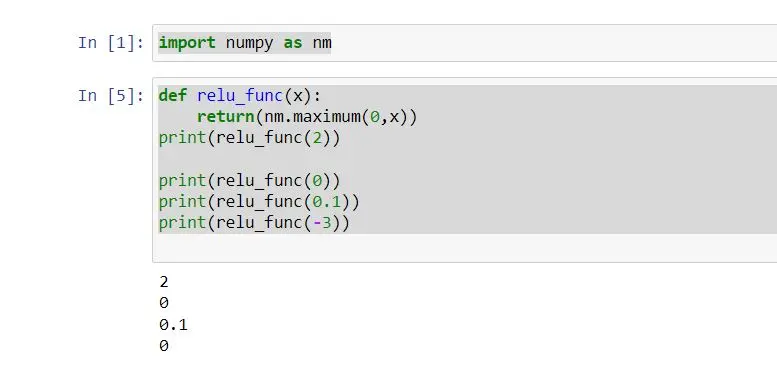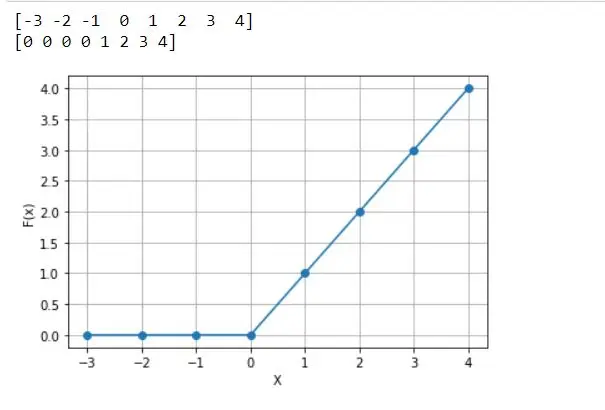# Derivative of ReLU Function in Python

Migel Hewage Nimesha Oct 10, 2023

ReLU stands for Rectified Linear Activation Function, which is the most popular alternative of activation function in the scope of deep learning. ReLU is a piece of the linear function that will output the input as the same if the input value is positive; if not, it will give the output zero.

This article indicates how to do a derivative of the ReLU function using the Python programming language.

## Implement ReLU Function in Python

As a mathematical function, we can define the ReLU function as follows:

``````f(x) = max(0,x)
``````

This function is linear about `x` and outputs zero for all negative values.

The following pseudo-code represents the ReLU function.

``````if input > 0:
return input
else:
return 0
``````

As the above pseudo-code, we can build our implementation of the ReLU function as follows:

``````import numpy as nm

def relu_func(x):
return nm.maximum(0, x)

print(relu_func(2))
print(relu_func(0))
print(relu_func(0.1))
print(relu_func(-3))
``````

Considering this example, it defined function `relu_func` with parameter `x`. This function returns output considering the ReLU function.

We passed a single integer at once to the `relu_func` function as an argument.

The `maximum()` function returns the highest value. If the integer is greater than 0, it will print the same integer as the input; if not, it prints zero.

So, the ReLU function we have implemented in the above code will work with any single integer; also with `numpy` arrays.

We can get the output as follows:## Derivative of ReLU Function in Python

The derivative of the ReLU function, otherwise, calls the gradient of the ReLu. The derivative of the function is the slope.

If we create a graph, for example, `y= ReLu(x)`, and `x` is greater than zero, the gradient is `1`.

If `x` is less than zero, the gradient is `0`. If `x = 0`, derivative does not be extant.

The mathematical derivative of the ReLu function can be defined as follows:

``````f'(x) = 1, x >= 0
= 0, x < 0
``````

We can apply the derivative of the ReLu function to the graph. So, let’s look at the following example.

``````# %matplotlib inline
import numpy as np
import matplotlib.pyplot as plt

# define relu function

def relu_func(z):
return np.maximum(0, z)

z = np.arange(-3, 5, 1)
print(z)
Y = relu_func(z)
print(Y)
plt.plot(z, Y, "o-")
plt.xlabel("X")
plt.ylabel("F(x)")
plt.grid()
``````

It’s defined as `relu_func` passing the `z` parameter considering the above code. Under this function, we return the `relu` function and the `z` variable defined to get the range of the x-axis.

Also defined `Y` variable defined to pass `relu_func` with a parameter. `relu_func(Z)` function computes the reLU for all `z` values.

Therefore, all negative values will denote a zero. The x-axis plot is `X` with the y-axis plot is `F(x)`.

The following plot is the output we get from the above code.As mentioned, derivative means the slope of the graph at a certain point. So, the slope of `x=1` is 1.

All the other points greater than 0 get a slope of 1. However, what is the slope of `x=-3`?

We can see there is no slope for that point.

All the other points less than 0 get slope none which means slope 0. So this is called the ReLu function’s derivative, built-in Python programming language.

## Conclusion

This article indicates how to implement the ReLu function in Python and mainly discusses how to implement ReLu derivative function. The ReLU function is used in deep learning frequently.

But it has some issues. For instance, if the input value is less than 0, the output will be 0.

Therefore, the neural network is unable to continue its some works. As a solution for this, it mostly uses the Leaky ReLU function.

Nimesha is a Full-stack Software Engineer for more than five years, he loves technology, as technology has the power to solve our many problems within just a minute. He have been contributing to various projects over the last 5+ years and working with almost all the so-called 03 tiers(DB, M-Tier, and Client). Recently, he has started working with DevOps technologies such as Azure administration, Kubernetes, Terraform automation, and Bash scripting as well.